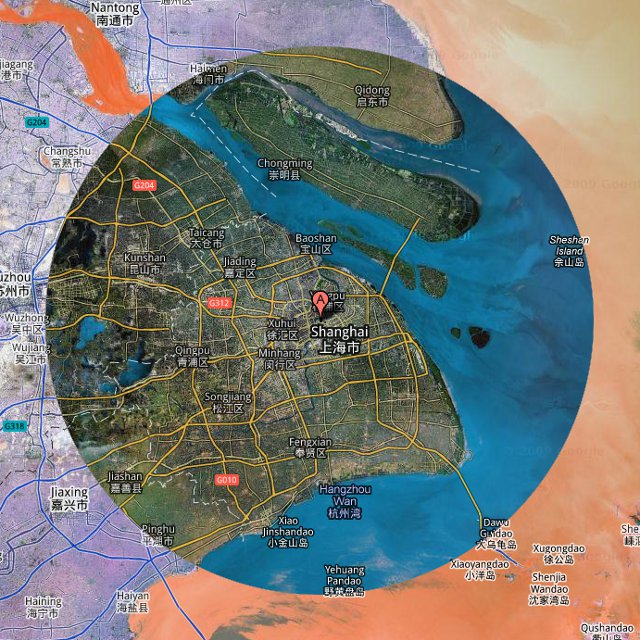# How far away is the horizon?It’s relatively easy to calculate how far away the horizon is if you know two things: the height of your eye above the ground, and how big the Earth is.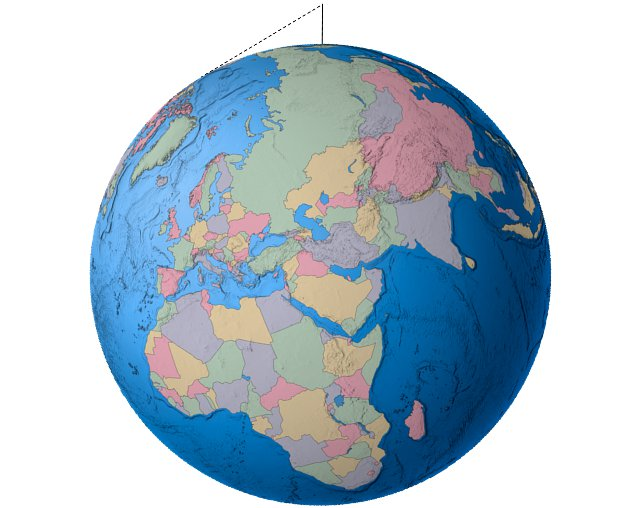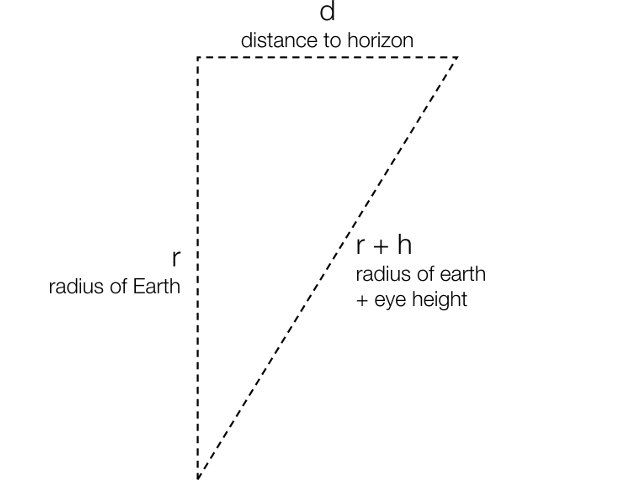Because we know r, the radius of the Earth, and we can measure h, the height of the eye above the ground, we can use Pythagorus’s theorem to calculate d, the distance to the horizon.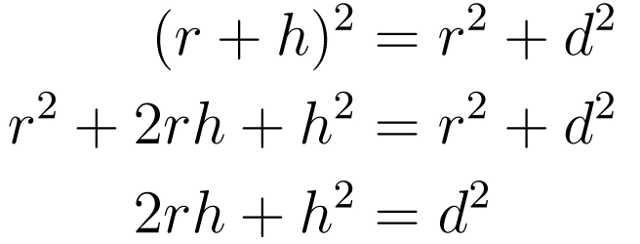Which gives an equation for the distance to the horizon d as a function of r and h:For the average person’s height of 1.62 m and the average radius of the Earth of 6367.5 km that gives a distance to the horizon of 4542 metres or 2.8 miles.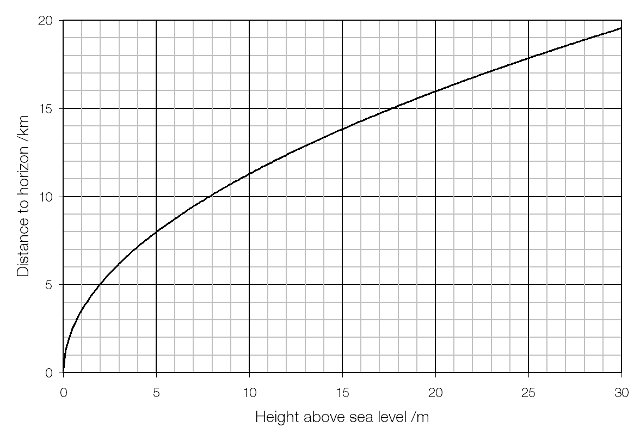From the world’s highest public observation deck, on the 100th floor of the Shanghai World Financial Centre at a height of 474 metres the distance to the horizon is 77.8 kilometres, giving a viewable area of nineteen billion square metres, over seven thousand square miles.